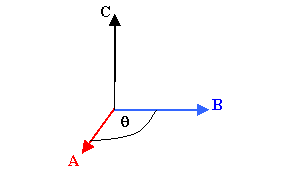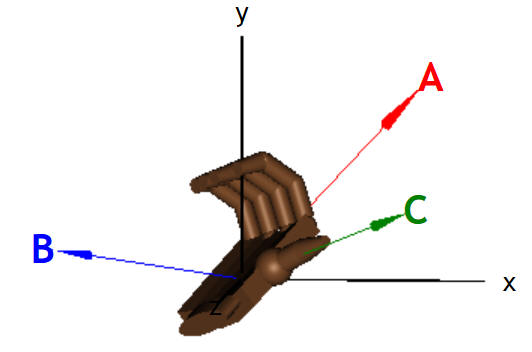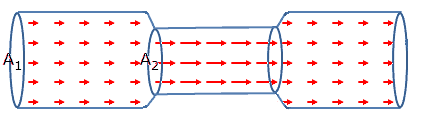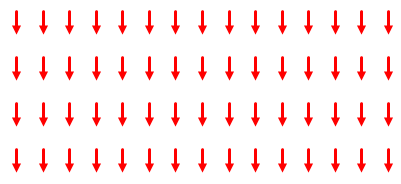## Vectors and vector fields

In physics, quantitative models are developed on the basis of measurements.  Measurements are made in standard increments, called units.  Without units, a measurement is meaningless.  Many quantities are specified by a magnitude (a number and the appropriate unit) and a direction in space.  Such quantities are called vector quantities.  Symbols that denote these vector quantities are bold letters, or normal letters with arrows drawn above.

Examples of vector quantities:

 displacement (d): d = 10 m north velocity (v): v = 3 m/s eastward acceleration (a) a = 6 m/s2 west force (F) F = 9 N up

To uniquely specify vector quantities, we need a reference point and reference lines, i.e. we need a coordinate system.  The most commonly used coordinate systems are rectangular, Cartesian coordinate systems.  Other widely used coordinate systems are cylindrical and spherical coordinate systems.

This semester we will study electromagnetic interactions.  To find the electric and magnetic fields produced by charged particles and the electric and magnetic forces acting on objects, we have to perform vector operations.

### Representing vectors

In Cartesian coordinates a vector is represented by its components along the axes of the coordinate system.
Example:  F = (Fx, Fy) = Fxi + Fyj  = 3 N i - 4 N j.
Here i and j are unit vectors.  Unit vectors have magnitude 1 and no units.  They are used as direction indicators.
(i, j, k point in the x-, y-, and z-direction, respectively.)

In the polar coordinates, in two dimensions, a vector is represented by its magnitude and the angle its direction makes with the x-axis.
Example:  F = (F, φ) = (5 N, 306.87o) = (5 N, -53.13o)
Cylindrical coordinates and spherical coordinates are two different extensions of polar coordinates to three dimensions.

To add or subtract physical vectors, they have to have the same units.  To find the sum of two physical vector quantities with the same units algebraically, we add the x, y, and z-components of the individual vectors.

#### Example:

In two dimensions, let vector A have components (3, 4) and vector B have components  (2, -3).  Let C = A + B be the sum of the two vectors.  Then the components of C are (3+2, 4+(-3) = (5, 1).
The magnitude of the vector C is C = (25 + 1)½ = 5.1, and the angle C makes with the x-axis is φ = tan-1(1/5) = 0.197 rad = 11.3o.
To subtract vector B from vector A we subtract the components of vector B from the components of vector A.

### Multiplying vectors

Vectors can be multiplied by a scalar (or number).  Multiplying  a vector by a scalar changes the magnitude of the vector, but leaves its direction unchanged.

#### Example:

F = (3 N, -4 N),  3F =  3*(3 N, -4 N) = (9 N, -12 N),
or
F = (F, φ) = (5 N, 323.13o),  3F = (15 N, 323.13o).

A vector can also be multiplied by another vector.  There are two different products of vectors.

The scalar product or dot product of two vectors A and B is a scalar quantity (a number with units) equal to the product of the magnitudes of the two vectors and the cosine of the smallest angle between them.
A∙B = ABcosθ.
In terms of the Cartesian components of the vectors A and B the scalar product is written as
A∙B = AxBx + AyBy + AzBz.
In one dimension, the scalar product is positive if the two vectors are parallel to each other, and it is negative if the two vectors are anti-parallel to each other, i.e. if they point in opposite directions.The vector product or cross product of two vectors A and B is defined as the vector C = A × B
The magnitude of C is C = AB sinθ, where θ is the smallest angle between the directions of the vectors A and B

C is perpendicular to both A and B, i.e. it is perpendicular to the plane that contains both A and B.
The direction of C can be found by using the right-hand rule.
Let the fingers of your right hand point in the direction of A.
Orient the palm of your hand so that, as you curl your fingers, you can sweep them over to point in the direction of B.
Your thumb points in the direction of C = A × B.If A and B are parallel or anti-parallel to each other, then C = A × B = 0, since sinθ = 0.
If A and B are perpendicular to each other, then sinθ = 1 and C has its maximum possible magnitude.

Please click on the image below for an animation!We can also find the Cartesian components of C = A × B in terms of the components of A and B.

Cx = AyBz - AzBy
Cy = AzBx - AxBz
Cz = AxBy - AyB

### Vector fields

If a vector can be assigned to each point in a subset of space, we have a vector field.

The velocity of a fluid, for example the velocity of water flowing through a pipe or down a drain, is a vector field.  The velocity field describes the motion of a fluid at every point.  The length of the flow velocity vector at any point is the flow speed.

### The gravitational field

Forces are vectors.  A force that we are familiar with is gravity.  The gravitational force is not a contact force.  It acts at a distance.  We introduce the concept of the gravitational field to explain this action at a distance.  Massive particles attract each other.  We say that massive particles produce gravitational fields and are acted on by gravitational fields.  The magnitude of the gravitational field produced by a massive object at a point P is the gravitational force per unit mass it exerts on another massive object located at that point.  The direction of the gravitational field is the direction of that force.  The gravitational field produced by a point mass always points towards the point mass and decreases proportional to the inverse square of the distance from the point mass.  Near the surface of Earth the gravitational field produced by Earth is nearly constant and has magnitude F/m = g = 9.8 m/s2.  Its direction is downward.
To find the total gravitational field at a point calculate the vector sum of the gravitational fields produced by all masses that do not produce negligibly small gravitational fields at that point.

### Graphical representations of vector fields

One way to graphically represent a vector field in two dimensions is by drawing arrows an a grid.  Set up a grid and find the magnitude and direction of the field vector at every grid point.  At each grid point draw an arrow with the tail anchored at the grid point and a length proportional to the magnitude of the vector in the direction of the field vector.  Most vector fields are three-dimensional.  But if the field has enough symmetry, a two dimensional representation can capture most of the information.

Examples:Velocity field of an ideal fluid in a pipe
The field exists everywhere in the pipe.  For the arrow representation we choose a plane that contains the symmetry axis of the pipe.  The vector field has rotational symmetry about this axis.
Continuity equation: A1v1 = A2v2
A2 = ½A1  -->  v2 = 2v1
The arrows in the narrower section of the pipe are twice as long as the arrows in the wider section.Gravitational field near the surface of Earth
The field exists everywhere in the space near the surface.  For the arrow representation we choose any vertical plane.
g = 9.8 m/s2 = constant, pointing downward.
All arrows have the same length.  Although we only draw arrows in one plane, a three dimensional representation also has arrows of the same length pointing downward everywhere.